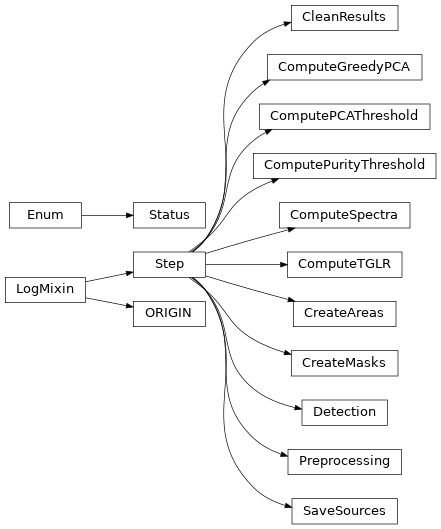# API¶

## Functions¶

 `Compute_GreedyPCA`(cube_in, test, thresO2, …) Function to compute greedy svd. `Compute_threshold_purity`(purity, …[, …]) Compute threshold values corresponding to a given purity. `Correlation_GLR_test`(cube, fsf, weights, …) Compute the cube of GLR test values with the given PSF and dictionary of spectral profiles. `add_tglr_stat`(src_table, lines_table, …) Add TGLR and STD detection statistics to the source and line table. `compute_deblended_segmap`(image[, npixels, …]) Compute segmentation map using photutils. `compute_local_max`(correl, correl_min, mask) Compute the local maxima of the maximum correlation and local maxima of minus the minimum correlation distribution. `compute_segmap_gauss`(data, pfa[, fwhm_fsf, bins]) Compute segmentation map from an image, using gaussian statistics. `compute_thresh_gaussfit`(data, pfa[, bins]) Compute a threshold with a gaussian fit of a distribution. `compute_true_purity`(cube_local_max, refcat) Compute the true purity using a reference catalog. `create_all_sources`(cat3_sources, cat3_lines, …) Create and save a MPDAF source file for each source. `create_masks`(line_table, source_table, …) Create the mask of each source. `create_source`(source_id, source_table, …) Create a MPDAF source. `estimation_line`(Cat1, raw, var, psf, wght, …) Compute the estimated emission line and the optimal coordinates for each detected lines in a spatio-spectral grid. `gen_source_mask`(source_id, ra, dec, lines, …) Generate a mask for the source segmenting the detection cube. `merge_similar_lines`(table, \*[, z_pix_threshold]) Merge and flag possibily duplicated lines. `purity_estimation`(cat, Pval, Pval_comp) Function to compute the estimated purity for each line. `spatial_segmentation`(Nx, Ny, NbSubcube[, start]) Compute indices to split spatially in NbSubcube x NbSubcube regions. `spatiospectral_merging`(tbl, tol_spat, tol_spec) Perform the spatial and spatio spectral merging. `unique_sources`(table) Return unique source positions in table.

## Classes¶

 `CleanResults`(orig, idx, param) Clean the various results. `ComputeGreedyPCA`(orig, idx, param) Loop on each area and compute the greedy PCA. `ComputePCAThreshold`(orig, idx, param) Loop on each area and estimate the threshold for the PCA. `ComputePurityThreshold`(orig, idx, param) Find the threshold for a given purity. `ComputeSpectra`(orig, idx, param) Compute the estimated emission line and the optimal coordinates. `ComputeTGLR`(orig, idx, param) Compute the cube of GLR test values. `CreateAreas`(orig, idx, param) Creation of areas to split the work. `CreateMasks`(orig, idx, param) Create source masks and sky masks. `Detection`(orig, idx, param) Detections on local maxima from correlation and std cube, and spatial-spectral merging in order to create the first catalog. `ORIGIN`(filename[, name, path, loglevel, …]) ORIGIN: detectiOn and extRactIon of Galaxy emIssion liNes `Preprocessing`(orig, idx, param) Preparation of the data for the following steps: `SaveSources`(orig, idx, param) Create the source file for each source. `Status` Step processing status. `Step`(orig, idx, param) Define a processing step.

## Class Inheritance Diagram¶